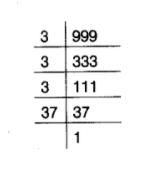# 999 is a perfect cube.

Question:

999 is a perfect cube.

Solution:

False

Resolving 999 into prime factors, we get

$999=3 \times 3 \times 3 \times 37$

Grouping the factors in triplets of equal factors, we get

$999=(3 \times 3 \times 3) \times 37$Clearly, in grouping, the factors in triplets of equal factors, we are left with one factor 37 .

Therefore, 999 is not a perfect cube.